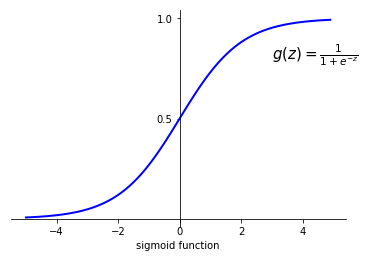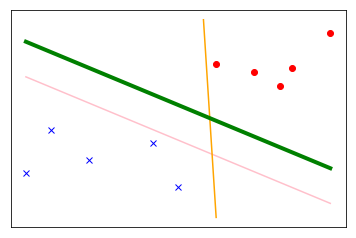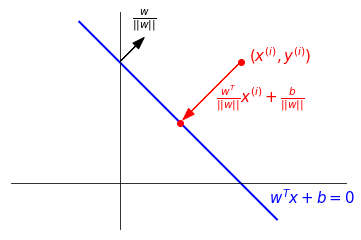## Intuition

In a binary classification problem, we can use logistic regression

$$h_\theta(x) = \frac{1}{1+e^{-\theta^T x}} = g(\theta^T x),$$

where $g$ is the sigmoid function with a figure of it below.Then given input $x$, the model predicts $1$ if and only if $\theta^x \ge 0$, in which case $h_\theta(x) = g(\theta^T x) \ge 0.5$; and it predicts $0$ if and only if $\theta^T x < 0$. Moreover, based on the shape of sigmoid function, if $\theta^T x >> 0$, we are very confident that $y=1$. Likewise, if $\theta^T x << 0$, we are very confident that $y=0$. Therefore, we hope that for the training set $\{(x^{(i)}, y^{(i)})\}_{i=1}^m$, we can find such a $\theta$ that $\theta^T x^{(i)} >> 0$ if $y^{(i)}=1$ and $\theta^T x^{(i)} << 0$ if $y^{(i)}=0$.

We can illustrate the idea using the figure below. Suppose we want to draw a line to separate the red dots and blue crosses. Then three lines in the figure serve this purpose.However, the bold green line is considered the best among these three lines since it is far away from both sides of data. This green line will meet our hope. With this intuition in mind, support vector machine is a model to find the bold green "line" for a classification problem.

## Margins

For now, we assume that the training set is linearly separable. Instead of $\{0, 1\}$, we assume that $y$ is valued in $\{\pm 1\}$, so the learning algorithm $h$ should output $1$ or $-1$ based on the input features $x$. We define a step function in place of sigmoid function:

$$\begin{equation} g(z) = \left\{ \begin{array}{ll} 1 & \text{ if } z \ge 0 \\ -1 & \text{ otherwise.} \end{array} \right. \label{eqn:step} \end{equation}$$

Suppose features $x \in \mathbb{R}^n$, since we assume the training set to be linearly separable, the learning algorithm takes the form

$$\begin{equation} h_{w, b}(x) = g(w^T x + b), \label{eqn:hypo} \end{equation}$$

where $w \in \mathbb{R}^n$ and $b \in \mathbb{R}$ is the parameters.

In logistic regression, we find the parameters via maximum likelihood estimate. However, in support vector machines, we want to reflect the intuition above to find a hyperplane determined by $(w, b)$ which is "far away" from both sides of the training data. To formulate this intuition precisely, we need a notion of margin.

Definition 1. The functional margin of a hyperplane $(w, b)$ with respect to a training sample $(x^{(i)}, y^{(i)})$ is $$\hat{\gamma}^{(i)} = y^{(i)}(w^T x^{(i)}+b).$$

The function margin of a hyperplane $(w, b)$ with respect to the entire training set is

$$\hat{\gamma} = \min_i \hat{\gamma}^{(i)}.$$

Remark. (1) The hyperplane $(w, b)$ in $\mathbb{R}^n$ is given by the equation $w^T x + b = 0$. Since $kw^T x + kb = 0$ determines the same hyperplane for any $k \in \mathbb{R}^\times$, the definition of functional margin depends not only on the position of the hyperplane, but also the parametrization of the hyperplane.

(2) Suppose $\hat{\gamma}^{(i)} > 0$. If $y^{(i)} = 1$, then $w^T x^{(i)} + b > 0$. By Equation ($\ref{eqn:hypo}$), $h_{w, b}(x^{(i)} = 1$. Similarly for the case $y^{(i)} = -1$. That is to say, that $\hat{\gamma}^{(i)} > 0$ means the i-th sample $(x^{(i)}, y^{(i)})$ is classified correctly with respect to $(w, b)$.

(3) The intuition section says, if $y^{(i)} = 1$, we want $w^T x^{(i)} + b >> 0$; if if $y^{(i)} = -1$, we want $w^T x^{(i)} + b << 0$. That amounts to require $\hat{\gamma}^{(i)} >> 0$. However, due to Remark (1), we can scale $\hat{\gamma}^{(i)}$ as much as we like, which is bad. To fix it, we introduce the following definition of geometric margins.

Definition 2. The geometric margin of a hyperplane $(w, b)$ with respect to a training sample $(x^{(i)}, y^{(i)})$ is $$\gamma^{(i)} = y^{(i)}\left(\frac{w^T}{||w||} x^{(i)}+\frac{b}{||w||}\right).$$

The geometric margin of a hyperplane $(w, b)$ with respect to the entire training set is

$$\gamma = \min_i \gamma^{(i)}.$$

Here, $||w|| = \sqrt{<w, w>} = \sqrt{w^T w}$ is the $L^2$ norm of $w$.

Remark. (1) $\frac{w^T}{||w||} x^{(i)}+\frac{b}{||w||}$ is signed distance from the sample point $(x^{(i)}, y^{(i)})$ to its projection on the hyperplace $w^T x + b = 0$. See the figure below. If $(x^{(i)}, y^{(i)})$ is classified correctly, then $\gamma^{(i)}$ is positive and it is the distance from the sample point to its projection.(2) Functional margins and geometric margins are related by

$$\begin{equation} \gamma^{(i)} = \frac{\hat{\gamma}^{(i)}}{||w||}. \label{eqn:margins} \end{equation}$$

## Maximum margin classifier

With the definitions of margins, we now are able to formulate the intuition precisely.

### Form 1

$$\max_{\gamma, w, b} \gamma,$$

subject to $y^{(i)}(w^T x^{(i)} + b) \ge \gamma$ and $||w||=1$.

Since we require $||w||=1$, functional margins and geometric margins are the same. In this form, the formulation means that the parameters $w^T$ and $b$ will maximize the worse case margins.

The scaling condition $||w||=1$ can be replaced by any other similar conditions. For example, we can set $|w_1| = 1$ or something like $w_1^2+w_2 = 17$, where $w = (w_1, w_2, ..., w_n)^T$.

### Form 2

$$\max_{\hat{\gamma}, w, b} \frac{\hat{\gamma}}{||w||},$$

such that $\hat{\gamma}^{(i)} = y^{(i)}(w^Tx+b) \ge \hat{\gamma}$.

According to Equation ($\ref{eqn:margins}$), Form 2 is the same as Form 1, but removing the condition $||w||=1$. These two forms are hard to optimize: Form 1 has a nonlinear boundary while Form 2 has a non-convex objective function.

### Form 3

Since $\hat{\gamma}$ is scalable (see remark (1) of Definition 1), we can then set $\hat{\gamma}=1$. Then Form 2 can be transformed into

$$\begin{equation} \min_{w, b} \frac{1}{2} ||w||^2, \label{eqn:form3} \end{equation}$$

subject to $y^{(i)}(w^T x^{(i)}+b) \ge 1$.

Form 3 has the advantages that the objective function is convex and the boundaries are linear. It is in this form that we will determine $w$ and $b$.

## Karush-Kuhn-Tucker conditions

We note that $f(w, b) = \frac{1}{2}||w||^2$ is a smooth function in $w$ and $b$. If we set

$$g_i(w, b) = 1 - y^{(i)}(w^T x^{(i)}+b),$$

then $g_i$ are also smooth in $w$ and $b$. Moreover, $g_i(x)$ are affine functions, so Karush-Kuhn-Tuchker can be applied without any other extra conditions. That is to say, if $w^\ast$ and $b^\ast$ is a local minimum for $f$, then it is necessary that there exist $\alpha_1, \dots, \alpha_m$, called the KKT multipliers, such that

Stationarity

$$\nabla f(w^\ast, b^\ast) + \sum_{i=1}^m \alpha_i \nabla g_i(w^\ast, b^\ast) = 0,$$

where $\nabla$ is with respect to $w$ and $b$.

Primal feasibility

$g_i(w^\ast, b^\ast) \le 0$, for $i = 1, \dots, m$.

Dual feasibility

$\alpha_i \ge 0$, for $i = 1, \dots, m$.

Complementary slackness

$\alpha_ig_i(w^\ast, b^\ast) = 0$, for $i = 1, \dots, m$.

Since the objective function $f$ is a nice quadratic function, the local minimum is indeed the absolute minimum.

We will say $g_i$ is a active constraint if $g_i(w^\ast, b^\ast) = 0$. By Complementary slackness, if $\alpha_i > 0$, then $g_i(w^\ast, b^\ast) = 0$.

$g_i$ is active amounts to $y^{(i)}((w\ast)^T x^{(i)}+b^\ast)=1$ by definition of $g_i$. It is the same as the functional margin of $(x^{(i)}, y^{(i)})$ with respect to the hyperplane $(w^\ast, b^\ast)$ is $1$. These special and important sample points $(x^{(i)}, y^{(i)})$ where $g_i$ is active are called suppoert vectors.

If we write $w = (w_1, \dots, w_n) \in \mathbb{R}^n$ and $x^{(i)} = (x^{(i)}_1, \dots, x^{(i)}_n)$, then we can expand $f$ to be

$$f(w, b) = \frac{1}{2}||w||^2 = \frac{1}{2}(w_1^2+\cdots+w_n^2),$$

and $g_i$ to be

$$g_i(w, b) = 1 - y^{(i)}(w_1x^{(i)}_1+\cdots+w_nx^{(i)}_n+b).$$

Then,

$$\begin{array}{ll} \frac{\partial f}{\partial w_j} = w_j & \frac{\partial f}{\partial 0} = 0 \\ \frac{\partial g_i}{\partial w_j} = -y^{(i)}x^{(i)}_j & \frac{\partial g_i}{\partial b} = -y^{(i)} \end{array}$$

Therefore, Stationarity becomes

$$\begin{equation} w = \sum_{i=1}^m \alpha_i y^{(i)} x^{(i)} \text{ and } \sum_{i=1}^m \alpha_i y^{(i)} = 0. \label{eqn:station} \end{equation}$$

## Primal problem and its dual

The Lagragian of the optimization problem in Form 3 is

$$\begin{equation} \begin{split} L(w, b, \alpha) &= f(w, b) + \sum_{i=1}^m \alpha_i g_i(w, b) \\ &= \frac{1}{2}||w||^2 + \sum_{i=1}^{m} \alpha_i(1-y^{(i)}(w^T x^{(i)}+b)) \end{split} \label{eqn:lagragian} \end{equation}$$

The primal problem attached to Form 3

$$\min_{w, b} \Theta_P(w, b),$$

where

$$\Theta_P(w, b) = \max_{\alpha, \alpha_i \ge 0} L(w, b, \alpha)$$

is an equivalent form of Form 3. This is because an observation that

$$\Theta_P(w, b) = \left\{\begin{array}{ll} \frac{1}{2}||w||^2 & \text{ if } g_i(w, b) \le 0 \\ \infty & \text{ otherwise.} \end{array}\right.$$

The dual problem of this primal problem is

$$\max_{\alpha, \alpha_i \ge 0} \Theta_D(\alpha),$$

where

$$\Theta_D(\alpha) = \min_{w, b} L(w, b, \alpha).$$

In general,

$$\max_{\alpha, \alpha_i \ge 0} \Theta_D(\alpha) \le \min_{w, b} \Theta_P(w, b).$$

If we assume $g_i$ are strictly feasible, i.e., there exist $w$ and $b$ such that $g_i(w, b) < 0$ for all $i=1, \dots, m$, then $\max_{\alpha, \alpha_i \ge 0} \Theta_D(\alpha) = \min_{w, b} \Theta_P(w, b)$ because $f(w, b)$ is convex. Since the primal problem is the same as the original problem in Form 3, $w, b$ and $\alpha_i$ satisfying Karush-Kuhn-Tucker(KKT) conditions above solve the primal problem, and therefore solve the dual problem also.

Suppose $w, b$ and $\alpha_i$ satisfy KKT condition for the optimization problem in Form 3, then Equations $(\ref{eqn:station})$ hold. Put them into $(\ref{eqn:lagragian})$,

$$\begin{split} L(w, b, \alpha) &= \frac{1}{2}<w, w> + \sum_{i=1}^{m} \alpha_i(1-y^{(i)}(<w, x^{(i)}>+b)) \\ &= \frac{1}{2} <\sum_{i=1}^m \alpha_i y^{(i)} x^{(i)}, \sum_{j=1}^m \alpha_j y^{(j)} x^{(j)}> + \sum_{i=1}^{m} \alpha_i(1-y^{(i)}(<\sum_{j=1}^m \alpha_j y^{(j)} x^{(j)}, x^{(i)}>+b)) \\ &= \sum_{i=1}\alpha_i - \frac{1}{2}y^{(i)}y^{(j)}<x^{(i)}, x^{(j)}>\alpha_i\alpha_j - b\sum_{i=1}^m \alpha_iy^{(i)} \\ &= \sum_{i=1}\alpha_i - \frac{1}{2}\sum_{i=1}^m \sum_{j=1}^m y^{(i)}y^{(j)}<x^{(i)}, x^{(j)}>\alpha_i\alpha_j \end{split}$$

If we set

$$\begin{equation} W(\alpha) = \sum_{i=1}\alpha_i - \frac{1}{2}\sum_{i=1}^m \sum_{j=1}^m y^{(i)}y^{(j)}<x^{(i)}, x^{(j)}>\alpha_i\alpha_j, \label{eqn:dual} \end{equation}$$

then the dual problem is equivalent to

$$\max_{\alpha} W(\alpha),$$

subject to $\alpha_i \ge 0$ and $\sum_{i=1}^m y^{(i)}\alpha_i = 0$.

If we can solve for $\alpha$ from this dual problem, then by part of Equation ($\ref{eqn:station}$), we can calculate $w$:

$$w = \sum_{i=1}^m \alpha_i y^{(i)} x^{(i)}.$$

And $b$ is calculated by

$$b = -\frac{\max_{i, y^{(i)}=-1}<w, x^{(i)}>+\min_{i, y^{(i)}=1} <w,x^{(i)}>}{2}.$$

Therefore, we get the model:

$$\begin{split} h_{w, b}(x) &= g(w^Tx+b) \\ &= g\left(\sum_{i=1}^m \alpha_i y^{(i)}<x^{(i)}, x>+b)\right) \end{split},$$

with $g$ being defined in Equation ($\ref{eqn:step}$).

## Kernel

The key of SVM is to maximize $W(\alpha)$ in Equation ($\ref{eqn:dual}$), which we will discuss later. But we note that the function $W(\alpha)$ only use $x^{(i)}$'s in inner products. We can replace inner product $<x^{(i)}, x^{(j)}>$ with some reasonable function $K(x^{(i)}, x^{(j)})$ without changing the algorithm.

Definition. A continuous function $K: \mathbb{R}^n \times \mathbb{R}^n \to \mathbb{R}$ is a Mercer kernel if there exists a continuous function $\phi: \mathbb{R}^n \to V$ for some real vector space $V$ such that

$$K(x, y) = <\phi(x), \phi(y)>,$$

where $<,>$ is a inner product in $V$.

Remark. Under the hood, Mercer kernel is still a inner product. So if we replace the inner products by a Mercer kernel, what it does is to replace the original features $x$ by the new features $\phi(x)$. Usually, it is more inexpensive to calculate $K(x, y)$ than to calculate $<\phi(x), \phi(y)>$ directly. There might be some cases where $V$ is infinite dimension while $K(x, y)$ is still feasible for calculation.

Example 1. Let $x, y \in \mathbb{R}^n$, consider

$$K(x, y) = (<x, y>)^2.$$

Then

$$\begin{split} K(x, y) &= (<x, y>)^2 = \left(\sum_{i=1}^nx_iy_i\right)\left(\sum_{j=1}^nx_jy_j\right) \\ &= \sum_{i, j}(x_ix_j)(y_iy_j) = <\phi(x), \phi(y)>, \end{split}$$

where $\phi: \mathbb{R}^n \to \mathbb{R}^{n^2}$ is

$$\phi(x) = (x_1x_1, \dots, x_ix_j, \dots, x_nx_n).$$

In this example, it takes $O(n)$ to calculate $K(x, y)$ while it takes $O(n^2)$ to calculate $<\phi(x), \phi(y)>$.

Similarly, for any integer $d \ge 1$, we can define a Mercer kernel

$$K(x, y) = (<x, y>)^d.$$

Example 2. Let $x, y \in \mathbb{R}^n$, define for a constant $c$

$$K(x, y) = (<x, y>+c)^2.$$

$K$ is still a Mercer kernel, with $\phi$ being

$$\phi(x) = (x_1x_1, \dots, x_nx_n, \sqrt{2c}x_1, \dots, \sqrt{2c}x_n, c).$$

Therefore, by using $K(x, y)$ in SVM, we use quadratic polynomials implicitly instead of linear functions. To generalize this, we can define for $d \ge 1$

$$K(x, y) = (<x, y>+c)^d.$$

Example 3. $\displaystyle K(x, y) = \exp\left(-\frac{||x-y||^2}{2\sigma^2}\right)$ for any $\sigma \ne 0$ is a Mercer kernel.

We don't have the tools to prove this is a Mercer kernel. To prove this, we need to construct Mercer kernels out of some given Mercer kernels. For example, we need that the product of two Mercer kernels is still a Mercer kernel, among other things.

Suppose $K(x, y)$ is a Mercer kernel, and $\phi$ is the attched function. Then for any set of points $\{x^{(1)}, \dots, x^{m}\}$, we define a $m \times m$ matrix $K$ where

$$K_{ij} = K(x^{(i)}, x^{(j)}) = <\phi(x^{(i)}), \phi(x^{(j)})>.$$

For any $z \in \mathbb{R}^m$,

$$\begin{split} z^T K z &= \sum_{i, j}z_iK_{ij}z_j \\ &= \sum_{i, j}z_iz_j<\phi(x^{(i)}), \phi(x^{(j)})> \\ &= <\sum_i z_i\phi(x^{(i)}), \sum_j z_j\phi(x^{(j)})> \\ &= ||\sum_i z_i \phi(x^{(i)})||^2 \ge 0. \end{split}$$

Therefore, the matrix $K$ is semi-positive definite. This necessary condition actually characterizes Mercer kernels.

Theorem (Mercer). Let $K: \mathbb{R}^n \times \mathbb{R}^n \to \mathbb{R}$ be a symmetric function. Then $K(x, y)$ is a Mercer kernel if and only if for any set of points $\{x^{(1)}, \dots, x^{(m)}\}$, the corresponding $m \times m$ matrix is semi-positive definite.

The idea of kernel is to map the original features/inputs into a higher dimensional (possibly infinite dimensional) feature space where we can separate classes using affine hyperplanes. The kernel trick is not only applied to SVM, but also be used in any algorithm involing inner product.

## $L_1$ norm soft margin SVM

Don't forget that the model given by the optimization problem ($\ref{eqn:form3}$) has a very strong assumption: the data are linearly separable. Even the data can be linearly separable, it might be a bad idea to do that, since it might be overfitting. To solve this problem, we relex the model, using the following optimization problem:

$$\min_{w, b, \xi} \frac{1}{2}||w||^2 + c\sum_{i=1}^m \xi_i,$$

subject to $y^{(i)}(w^Tx^{(i)}+b) \ge 1 - \xi_i$ and $\xi_i \ge 0$ for $i=1, \dots, m$, where $c$ is some fixed constant.

By introducing the parameters $\xi_i$, we allow the model to be error tolerent: If $y^{(i)}(w^Tx^{(i)}+b) > 0$, then the model classifies $(x^{(i)}, y^{(i)})$ correctly. Without $\xi_i$, $y^{(i)}(w^Tx^{(i)}+b) \ge 1$ requires the model to classify every point correctly. But in the new optimization problem, $\xi_i > 1$ allows $y^{(i)}(w^Tx^{(i)}+b)$ to be negative, meaning that the model accepts errors. Adding $\xi_i$ to the objective function is the reflection of penalty of classifying it incorrectly.

Let $g_i(w, b, \xi) = 1-\xi_i-y^{(i)}(w^Tx^{(i)}+b)$ and $h_i(w, b, \xi) = -\xi_i$ for $i=1, \dots, m$. There are KKT multiplers $\alpha_1, \dots, \alpha_m, \beta_1, \dots, \beta_m$, such that

Stationarity

$$\nabla f(w, b, \xi) + \sum_{i=1}^m \alpha_i \nabla g_i(w, b, \xi) + \sum_{i=1}^m \beta_i \nabla h_i(w, b, \xi)= 0,$$

where $\nabla$ is with respect to $w, b$ and $\xi$. If we put in the formulas for $f, g_i$ and $h_i$, we get

$$\begin{equation} \begin{split} & w = \sum_{i=1}^m \alpha_i y^{(i)} x^{(i)} \\ & \sum_{i=1}^m \alpha_i y^{(i)} = 0 \\ & \alpha_i + \beta_i = c \text{ for }i = 1, \dots, m \end{split} \label{eqn:station2} \end{equation}$$

Primal feasibility

$g_i(w, b, \xi) \le 0$ and $h_i(w, b, \xi) \le 0$, for $i = 1, \dots, m$.

Dual feasibility

$\alpha_i \ge 0$ and $\beta_i \ge 0$, for $i = 1, \dots, m$.

Complementary slackness

$\alpha_ig_i(w, b, \xi) = 0$ and $\beta_i h_i(w, b, \xi) = 0$, for $i = 1, \dots, m$.

The Lagragian for the problem is

$$L(w, b, \xi, \alpha, \beta) = f(w, b, \xi) + \sum_{i=1}^m \alpha_i g_i(w, b, \xi) + \sum_{i=1}^m \beta_i h_i(w, b, \xi).$$

Using Stationarity ($\ref{eqn:station2}$),

$$\begin{split} L(w, b, \xi, \alpha, \beta) &= \frac{1}{2}<w, w> + c\sum_{i=1}^m \xi_i + \sum_{i=1}^{m} \alpha_i(1-\xi_i - y^{(i)}(<w, x^{(i)}>+b)) - \sum_{i=1}^m \beta_i \xi_i \\ &= \sum_{i=1}\alpha_i - \frac{1}{2}y^{(i)}y^{(j)}<x^{(i)}, x^{(j)}>\alpha_i\alpha_j - b\sum_{i=1}^m \alpha_iy^{(i)} + \sum_{i=1}^m (c-\alpha_i-\beta_i) \xi_i\\ &= \sum_{i=1}\alpha_i - \frac{1}{2}\sum_{i=1}^m \sum_{j=1}^m y^{(i)}y^{(j)}<x^{(i)}, x^{(j)}>\alpha_i\alpha_j \end{split}$$

Then the dual problem is to maximize

$$\begin{equation} W(\alpha) = \sum_{i=1}\alpha_i - \frac{1}{2}\sum_{i=1}^m \sum_{j=1}^m y^{(i)}y^{(j)}<x^{(i)}, x^{(j)}>\alpha_i\alpha_j, \label{eqn:dual2} \end{equation}$$

suject to $\sum_i\alpha_i y^{(i)} = 0, \alpha_i \ge 0$, and $\beta_i \ge 0$ which is the same as $\alpha_i \le c$ since $\alpha_i+\beta_i = c$.

## SMO algorithm

The last thing left is to solve optimization problem ($\ref{eqn:dual2}$). It can be solved using a modified version of coordinate descent algorithm, which is called sequential minimal optimization.

The core idea of SMO is to choose a pair $(\alpha_i, \alpha_j)$ in each iteration, and treat all other $\alpha_k$ as constants. The original problem ($\ref{eqn:dual2}$) becomes a problem only involving two varibles, which can be solved easily.

But in each iteration, which pair $(\alpha_i, \alpha_j)$ should we choose? And when should the algorithm terminate? To answer this question, we need to go back to the KKT conditions. To derive the dual problem ($\ref{eqn:dual2}$), we only use stationarity. There will be some implications from other conditions. For example, if $\alpha_i=0$, then from stationarity ($\ref{eqn:station2}$), $\beta_i = c-\alpha_i=c$. Now by complementary slackness that $\beta_ih_i=0$, we must have $\xi_i = 0.$ By primal feasibility, we have $g_i \le 0$, i.e.,

$$y^{(i)}(w^Tx^{(i)}+b) \ge 1.$$

Similarly, if $\alpha_i=c$, then $y^{(i)}(w^Tx^{(i)}+b) \le 1$; if $0 < \alpha_i < 1$, then $y^{(i)}(w^Tx^{(i)}+b) = 1$. Put them together,

$$\begin{equation} \begin{array}{llll} & \alpha_i = 0 & \implies & y^{(i)}(w^Tx^{(i)}+b) \ge 1 \\ & 0 < \alpha_i < c & \implies & y^{(i)}(w^Tx^{(i)}+b) = 1 \\ & \alpha_i = c & \implies & y^{(i)}(w^Tx^{(i)}+b) \le 1 \end{array} \label{eqn:conv} \end{equation}$$

Once we know the values of $\alpha_i$ for all $i=1, \dots, m$, we can calculate $w$ by

$$w = \sum_{i=1}^m \alpha_iy^{(i)}x^{(i)},$$

and $b$ by

$$b = -\frac{\max_{i, y^{(i)}=-1}<w, x^{(i)}>+\min_{i, y^{(i)}=1} <w,x^{(i)}>}{2},$$

where max and min are over those $i$ such that $(x^{(i)}, y^{(i)})$ are classfied correctly.

So in each iteration, we can try to find out one $\alpha_i$ such that it violates Equations $(\ref{eqn:conv})$ and pair it with anohter $\alpha_j$. And the algorithm terminates when all $\alpha_i$ satisfy Equations $(\ref{eqn:conv})$ within some error.